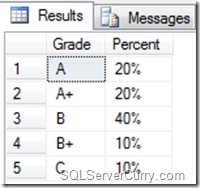### Calculate Percentage in SQL Server

The following T-SQL script shows a simple example to calculate Percentage in SQL Server. We will be using a table which contains Grades and calculate the percentage weight of each grade, for the entire classroom

SAMPLE DATA

CREATE TABLE #ClassRoom(ID INT IDENTITY(1,1), Grade char(2) NULL);
GO
-- Code by SqlServerCurry.com
INSERT INTO #ClassRoom Values ('A');
INSERT INTO #ClassRoom Values ('B');
INSERT INTO #ClassRoom Values ('B+');
INSERT INTO #ClassRoom Values ('B');
INSERT INTO #ClassRoom Values ('A');
INSERT INTO #ClassRoom Values ('A+');
INSERT INTO #ClassRoom Values ('B');
INSERT INTO #ClassRoom Values ('B');
INSERT INTO #ClassRoom Values ('A+');
INSERT INTO #ClassRoom Values ('C');

QUERY

CONVERT(varchar, 100 * count(*) / tot,1) + '%' as 'Percent'
FROM #ClassRoom,
(SELECT COUNT(*) as tot FROM #ClassRoom) x

The CONVERT function formats the percentage figure so that you get the result in a percentage format.

OUTPUT